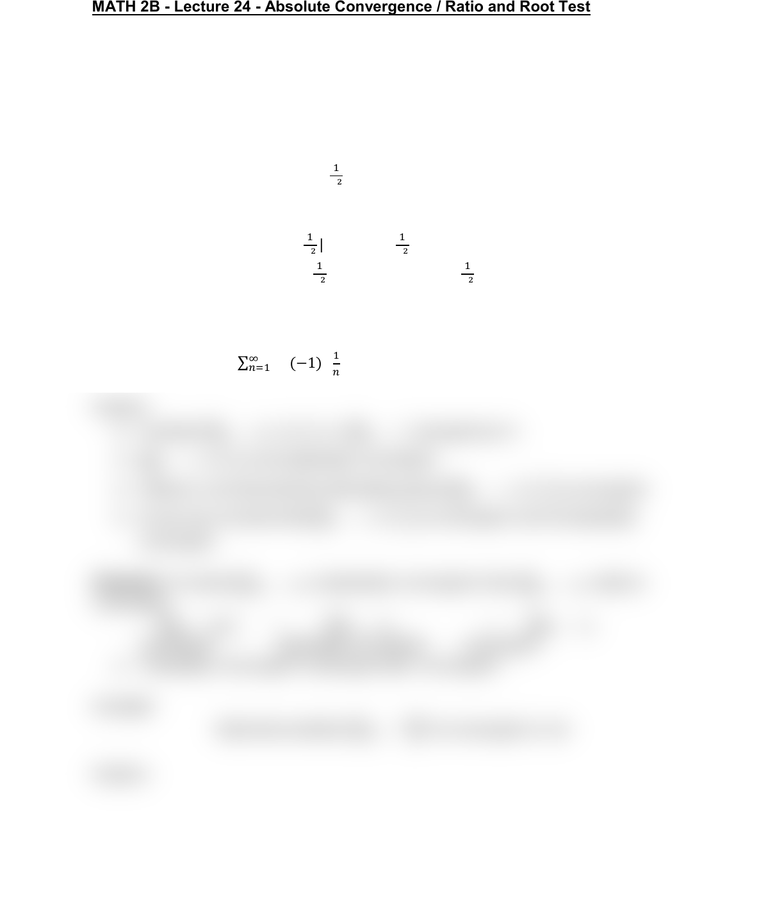Class Notes (1,100,000)
US (460,000)
UC-Irvine (10,000)
MATH (1,000)
MATH 2B (600)
Lecture 24

# MATH 2B Lecture Notes - Lecture 24: Ratio TestPremium

Department
Mathematics
Course Code
MATH 2B
Professor
ERJAEE, G.
Lecture
24

This preview shows half of the first page. to view the full 2 pages of the document.03/01/2019
MATH 2B - Lecture 24 - Absolute Convergence / Ratio and Root Test
Given a series
 , we can consider the corresponding series
  
A series
 is called absolutely convergent if the series of absolute values
  is convergent
Example:
 
absolutely convergent or not?
Solution:
Consider
 

convergent (p=2)
Therefore,
 
 
convergent absolutely convergent
Example:
absolutely convergent or not?
Solution:
Consider
divergent (p=1)
is not absolutely convergent
However, we know that the alternating series
is convergent
So we can conclude that
is convergent, but not absolutely
convergent
Theorem: If a series
is absolutely convergent, then
must be
convergent
convergent absolutely convergent convergent
“Absolutely convergent” is stronger than “convergent”
Example:
Determine whether

is convergent or not
Solution: